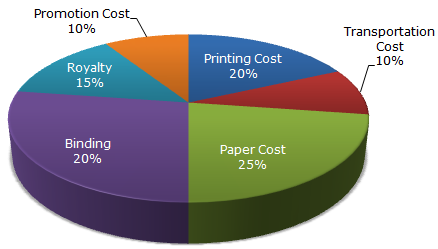# Data Interpretation - Pie Charts - Discussion

The following pie-chart shows the percentage distribution of the expenditure incurred in publishing a book. Study the pie-chart and the answer the questions based on it.

Various Expenditures (in percentage) Incurred in Publishing a Book5.

Royalty on the book is less than the printing cost by:

[A]. 5%
[B].
 33 1 % 5
[C]. 20%
[D]. 25%

Explanation:

Printing Cost of book = 20% of C.P.

Royalty on book = 15% of C.P.

Difference = (20% of C.P.) - (15% of C.P) = 5% of C.P.Percentage difference =Difference x 100% Printing Cost =5% of C.P. x 100% = 25%. Printing Cost

 Saurah said: (Jan 5, 2011) Sometimes percentage difference is only the difference between the percentage and in some case it is divided by something as done in this question.

 Aneesh said: (Jan 31, 2011) Is there any standard formula for percentage difference?

 Suzan said: (Jun 27, 2011) Can anyone elaborate it ?

 Raju said: (Aug 9, 2011) C. P means?

 Surendra Choudhary said: (Aug 10, 2011) Sometimes percentage difference is only the difference between the percentage and in some case it is divided by something as done in this question. How we judge which type of problem is this. ? Please suggest me

 Anand said: (Aug 25, 2011) C.P means Cost Price

 Jassi said: (Sep 9, 2011) Here for better understanding, Lets we can assume C.P.=100rs now, 5% of C.P. is 5rs and printing cost is 20% of C.P.=20rs Now you can calculate: % diff=5% of C.P./20% of C.P*100=5/20*100=25% answer.

 Rishant Jain said: (Dec 22, 2011) I am not able to understand why they have divided difference by printing cost not by royalty cost?

 Neelam said: (Jan 20, 2012) Yes. Rishant is correct. It should have been divided by the royalty.

 Fazokhan said: (Nov 22, 2012) Divide by printing cost because they are comparing the cost of royality with respect to printing cost.

 Mahendra Singh said: (Apr 26, 2013) 15*100/20=75% that means 15 is 25% less than 20.

 Medha said: (May 29, 2014) If A is x% of B then can we say A is (100-x) % less than B? Can we generalize this fact?

 Mani said: (Jun 16, 2014) Ya there is formula for difference as (Diff/Initial value) *100.

 Kasinath @Hyd said: (Aug 20, 2014) Royalty= 15. Printing Cost= 20. (20-15)*100/20=25 or (15-20)*100/20=25. Here we used divided by 20 just bcoz to get "LESS" value {always divide by bigger value i.e., 20(>15)} We used 100 to get %value.

 Jaimini said: (Sep 1, 2014) How can we know that we have to solve this with respect to printing price?

 Crazysatish said: (Sep 4, 2014) If question asked how much less than formula, = (diff/big number)*100. (5/20)*100 = 25%. If how much more is asked then =(diff/small no)*100. (5/15)*100= 100/3.

 Raynia said: (May 17, 2015) Why do you have to find C.P and stuff you could just directly just do the damn chart.

 Kiran said: (Sep 15, 2016) It's simple. 20 - 15/20 * 100 = 25.

 Ujwal said: (Feb 5, 2017) @Neelam. If the question asked for how more % the printing cost was, it would be divided by royalty!

 Esk said: (Feb 6, 2017) Ans should be 5%. Say price is 20,000. So royalty:3000. Printing comes out to be:4000. And their difference is 1000 which is 5% of 20,000. So, the answer must be 5% rather than 25%.

 Mano said: (Sep 20, 2017) If there is no fixed amount then we can consider it as 100 or any amount. I can't get in this case how is it 25%?

 Vinny said: (Jan 12, 2018) I can't get it. Please explain me.

 Ranjay Kumar Mehta said: (Jan 13, 2018) It's simple. 20-15=5. 5*100/20=25.

 Tej said: (Jun 2, 2018) Explain.

 Reshma said: (Aug 22, 2018) Yes, correct @Crazysatish.

 Kritika said: (Oct 4, 2018) LET CP 100. royalty 15. printing cost 20, difference/printing cost * 100, = 5/20 * 100 , = 25%.

 Sri said: (Jun 20, 2019) Not getting this, please explain it clearly.

 Swetha said: (Sep 24, 2019) Why we divide by printing cost?

 Shubham said: (Sep 26, 2019) Simply, let us consider the C.P of the book is Rs 100. Now, Royalty on the book is Rs 5 less than Printing cost. so as to calculate the percentage decrease with printing price it is, 5/20 * 100 = 25% less.

 Shakti said: (Nov 25, 2019) (20 - 15/20 )* 100 = 25.

 Gokul said: (Jun 23, 2020) @rishant. As the question was asked to find the %difference for royalty as of printing cost, we divide the difference with printing cost.

 Jagadeesh0074 said: (Apr 4, 2021) Royalty is 15% and printing cost is 20%. Lets assume the cost price (C.P) of book is 100, then royalty = 15 , printing cost = 20. This means that royalty is 75% of printing cost. Thus the % difference between royalty and printing cost is (100% - 75%) = 25%.